Processing ......FreeComputerBooks.com Links to Free Computer, Mathematics, Technical Books all over the World

Data Science and Data Engineering
Related Book Categories:
 Data Analysis and Data MiningBig Data Python Programming Non-relational/NoSQL Databases Statistics and SAS Programming Database Theory and Systems Data Visualization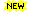Machine Learning Deep Learning & Neural Networks Data Storage, Data Warehouse, Data Lake, etc. R Programming Information Retrieval & Search Engines
•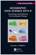Geographic Data Science with R: Visualizing and Analyzing

This book provides a series of tutorials aimed at teaching good practices for using Time Series and geospatial data to address topics related to environmental change. It is based on the R language and aims at data scientists.

•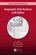Geographic Data Science with Python (Sergio Rey, et al.)

Provides the tools, the methods, and the theory to meet the challenges of contemporary data science applied to geographic problems and data, by using geographical and computational reasoning to unlock new insights hidden within data.

•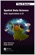Spatial Data Science: With Applications in R (Edzer Pebesma, et al)

Introduces and explains the concepts underlying Spatial Data, gives a detailed explanation of the core spatial software packages for R: sf for simple feature access, and stars for raster and vector data cubes – array data with spatial and temporal dimensions.

•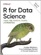R for Data Science: Visualize, Model, Transform, Tidy, Import

This book teaches you how to do data science with R: You'll learn how to get your data into R, get it into the most useful structure, transform it, visualize it and model it, how data science can help you work with the uncertainty and capture the opportunities.

•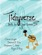Tidyverse Skills for Data Science (Carrie Wright, et al)

Intended for data scientists with some familiarity with the R programming language who are seeking to do data science using the Tidyverse family of packages, covers the entire life cycle of a data science project and presents specific tidy tools for each stage.

•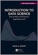Introduction to Data Science (Rafael A. Irizarry)

Introduces concepts and skills that can help tackling real-world data analysis challenges. Covers concepts from probability, statistical inference, linear regression, and machine learning. Helps developing skills such as R programming, data wrangling, etc.

•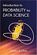Introduction to Probability for Data Science (Stanley Chan)

This book is an introductory textbook in undergraduate probability in the context of data science to emphasize the inseparability between data (computing) and probability (theory) in our time, with examples in both MATLAB and Python.

•Data Science at the Command Line, 2nd Ed. (Jeroen Janssens)

This hands-on guide demonstrates how the flexibility of the command line can help you become a more efficient and productive data scientist. Learn how to combine small, yet powerful, command-line tools to quickly obtain, scrub, explore, and model your data.

•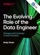The Evolving Role of the Data Engineer (Andy Oram)

If you're pursuing a career in data engineering or looking for ways to adapt your enterprise to the world of big data, this report shares the knowledge you need to find your way forward.

•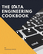Data Engineering Cookbook: The Plumbing of Data Science

This is a practical and comprehensive guide. You will learn the basics of data engineering. Then you will learn the technologies and frameworks required to build data pipelines to work with large datasets.

•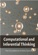Computational and Inferential: The Foundations of Data Science

Step by step, you'll learn how to leverage algorithmic thinking and the power of code, gain intuition about the power and limitations of current machine learning methods, and effectively apply them to real business problems.

•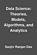Data Science: Theories, Models, Algorithms, and Analytics

It provides a bucket full of information regarding Data Science, covers a wide variety of sections by giving access to theories, data science algorithms, tools and analytics. You'll explore the right approach to best practices to guide you along the way.

•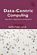A Data-Centric Introduction to Computing (Kathi Fisler, et al)

This book is an introduction to computer science. It will teach you to program, and do so in ways that are of practical value and importance. It uses a data-centric approach: data centric = data science + data structures.

•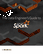The Data Engineer's Guide to Apache Spark (Databricks)

This book is for data engineers looking to leverage the immense growth of Apache Spark to build faster and more reliable data pipelines. It leverages Spark's amazing speed, scalability, simplicity, and versatility to build practical Big Data solutions.

•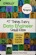97 Things Every Data Engineer Should Know (Tobias Macey)

With this in-depth book, current and aspiring engineers will learn powerful real-world best practices for managing data big and small. Experts share their experiences and lessons learned for overcoming a variety of specific and often nagging challenges.

•Python Data Science Handbook: Essential Tools (Jake VanderPlas)

Several resources exist for individual pieces of this data science stack, but only with the Python Data Science Handbook do you get them all - IPython, NumPy, Pandas, Matplotlib, Scikit-Learn, and other related tools.

•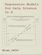Regression Models for Data Science in R (Brian Caffo)

The book gives a rigorous treatment of the elementary concepts of regression models from a practical perspective. The ideal reader for this book will be quantitatively literate and has a basic understanding of statistical concepts and R programming.

•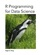R Programming for Data Science (Roger D. Peng)

This book is about the fundamentals of R programming. Get started with the basics of the language, learn how to manipulate datasets, how to write functions, and how to debug and optimize code. You will have a solid foundation on data science toolbox.

•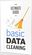The Ultimate Guide to Effective Data Cleaning

With this in-depth book, current and aspiring engineers will learn powerful real-world best practices for managing data big and small. Experts share their experiences and lessons learned for overcoming a variety of specific and often nagging challenges.

•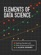Elements of Data Science (Allen B. Downey)

This book is an introduction to data science for people with no programming experience. The goal is to present a small, powerful subset of Python that allows you to do real work in data science as quickly as possible.

•Statistical Inference: Algorithms, Evidence, and Data Science

A masterful guide to how the inferential bases of classical statistics can provide a principled disciplinary frame for the data science of the twenty-first century. Every aspiring data scientist should carefully study this book, use it as a reference.

•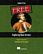Exploring Data Science (Nina Zumel, et al)

This book introduces readers to various areas in data science and explains which methodologies work best for each, with practical examples in R, Python, and other languages.

•Introduction to Data Science (Jeffrey Stanton)

This book provides non-technical readers with a gentle introduction to essential concepts and activities of data science. For more technical readers, the book provides explanations and code for a range of interesting applications using the open source R language for statistical computing and graphics.

•The Data Science Handbook: Advice and Insights

This book covers the essential exploratory techniques for summarizing data with R. These techniques are typically applied before formal modeling commences and can help inform the development of more complex statistical models.

•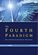The Fourth Paradigm: Data-Intensive Scientific Discovery

This book presents the first broad look at the rapidly emerging field of data-intensive science, with the goal of influencing the worldwide scientific and computing research communities and inspiring the next generation of scientists.

•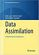Data Assimilation: A Mathematical Introduction (Kody Law, et al)

This book provides a systematic treatment of the mathematical underpinnings of work in data assimilation, covering both theoretical and computational approaches. Specifically the authors develop a unified mathematical framework of Bayesian formulation.

•School of Data Handbook

This textbook will provide the detail and background theory to support the Data Science courses and challenges. It will guide you through the key stages of a data project. These stages can be thought of as a pipeline, or a process.

•Machine Learning for Data Streams: Practical Examples in MOA

This book presents algorithms and techniques used in data stream mining and real-time analytics. Taking a hands-on approach, it demonstrates the techniques using MOA (Massive Online Analysis), a popular, freely available open-source software framework, allowing readers to try out the techniques after reading the explanations.

•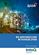Big Data Processing with Apache Spark (Srini Penchikala)

Learn about the Apache Spark framework and develop Spark programs for use cases in big-data analysis. It covers all the libraries that are part of Spark ecosystem, which includes Spark Core, Spark SQL, Spark Streaming, Spark MLlib, and Spark GraphX.

•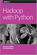Hadoop with Python (Zachary Radtka, et al)

This book takes you through the basic concepts behind Hadoop, MapReduce, Pig, and Spark. Then, through multiple examples and use cases, you'll learn how to work with these technologies by applying various Python tools.

•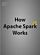Mastering Apache Spark 2.0 (Jacek Laskowski)

This book introduces Apache Spark, the open source cluster computing system that makes data analytics fast to write and fast to run. With Spark, you can tackle big datasets quickly through simple APIs in Python, Java, and Scala.

•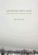Modeling with Data: Tools and Techniques for Scientific Computing

Modeling with Data fully explains how to execute computationally intensive analyses on very large data sets, showing readers how to determine the best methods for solving a variety of different problems, etc..

•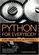Exploring Data in Python 3

This book is designed to introduce students to programming and software development through the lens of exploring data. You can think of the Python programming language as your tool to solve data problems that are beyond the capability of a spreadsheet.

•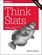Think Stats, 2nd Edition: Exploratory Data Analysis in Python

This concise introduction shows you how to perform statistical analysis computationally, rather than mathematically, with programs written in Python.

•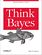O'Reilly® Think Bayes: Bayesian Statistics Made Simple

An introduction to Bayesian statistics using computational methods and Python. You'll learn how to solve statistical problems with Python code instead of mathematical notation, and use discrete probability distributions instead of continuous mathematics.

•The Global Impact of Open Data: Key Findings from Case Studies

Open data has spurred economic innovation, social transformation, etc. This book presents detailed case studies of open data projects throughout the world, along with in-depth analysis of what works and what doesn't.

Book Categories
 :All CategoriesTop Free BooksRecent BooksMiscellaneous BooksComputer EngineeringComputer LanguagesComputer ScienceData Science/DatabasesJava and Java EE (J2EE)Linux and UnixMathematicsMicrosoft and .NETMobile ComputingNetworking and CommunicationsSoftware EngineeringSpecial TopicsWeb Programming
Other Categories